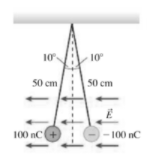# Problem: The identical small spheres shown in the figure on the right are charged to +100 nC and -100 nC. They hang as shown in a 100,000 N/C electric field. What is the mass of each sphere?

###### FREE Expert Solution

The force exerted by an external electric field on the charged sphere is:

$\overline{){{\mathbf{F}}}_{{\mathbf{E}}}{\mathbf{=}}{\mathbf{|}}{\mathbf{q}}{\mathbf{|}}{\mathbf{E}}}$, where E is the electric field and q is the charge.

Coulomb's law:

$\overline{){\mathbf{F}}{\mathbf{=}}\frac{\mathbf{k}\mathbf{|}{\mathbf{q}}_{\mathbf{1}}\mathbf{|}\mathbf{|}{\mathbf{q}}_{\mathbf{2}}\mathbf{|}}{{\mathbf{r}}^{\mathbf{2}}}}$

From the figure given, the gravitational force, mg, acts in the downward direction.

Tension, T acts in the upward direction along the rope.

The x-component of T is in the negative x-direction and is given by:

Tx = Tsin(10°)

The y-component of T along the positive y-direction is:

Ty = Tcos(10°)

Since the spheres have opposite charges, the Coulombic force between them is attractive.

The force of attraction F on the second charge is along the x-direction.

The electric field is along the x-direction.

93% (480 ratings)###### Problem Details

The identical small spheres shown in the figure on the right are charged to +100 nC and -100 nC. They hang as shown in a 100,000 N/C electric field. What is the mass of each sphere?Frequently Asked Questions

What scientific concept do you need to know in order to solve this problem?

Our tutors have indicated that to solve this problem you will need to apply the Coulomb's Law (Electric Force) concept. You can view video lessons to learn Coulomb's Law (Electric Force). Or if you need more Coulomb's Law (Electric Force) practice, you can also practice Coulomb's Law (Electric Force) practice problems.